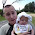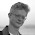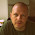Thursday, September 6, 2012

A limit to my understanding

One of the many things I find hard to understand about quantum many-body theory is the fact that the order in which you take the limits of functions really does matter. I fear I was brainwashed by too many calculus classes which always considered well defined analytical functions!

Two significant and profound examples concern the theory of superconductivity and the the thermoelectric effect.

Example one: electromagnetic response of a superconductor

Consider the frequency and wavevector dependence of the current-current correlation function, denoted Lambda(q,omega) below, where q is the wavevector and omega the frequency. The q to 0 limit can also be viewed as taking the thermodynamic limit.

The following equations are taken from an important 1993 paper Insulator, metal, or superconductor: the criteria by Doug Scalapino, Steve White, and Shoucheng Zhang
The first equation defines the superfluid density D_s, and the last one the Drude weight D. The two quantities are equal in a BCS superconductor but are not equal in a non-superconducting metal or in unconventional superconductors such as cuprates or organic charge transfer salts.

The second equation is required by gauge invariance. Comparing to the first equation we see that one gets a different answer depending on the order in which q_x (parallel to the current) and q_y goes to zero.

Example two: thermoelectric response

A very elegant 2010 paper Kelvin formula for thermopower, Michael Peterson and Sriram Shastry considered the significance of taking limits in different orders. They started with the exact Kubo expression for the frequency and wavevector dependent thermoelectric response S(q,omega).

The correct value for the Seebeck coefficient is obtained by first taking the q to 0 (i.e. thermodynamic limit of infinite system size) and then taking the static (omega to 0) limit.  However, if one reverses the order of these two limits  S(q,omega) reduces to Kelvin's (1854) formula which gives the Seebeck coefficient (n.b. a transport property) in terms of purely thermodynamical variables, i.e. S is proportional to the derivative of the chemical potential with respect to temperature. This formula is only approximate, but is very useful particularly for getting magnitudes and trends.

The slide below from a 2012 talk by Shastry nicely summarises the above.
Can someone give other examples?

1.Mahan discusses in his Kubo formulae section (ch 3 from memory) that when taking the dc conductivity, you calculate the finite \omega, finite q current-current correlation function, and then you must first take q->0, then \omega->0.

Done the wrong way, ie first taking \omega->0 enforces a static electric field. In this case no current will flow. Taking first the q->0, one gets a finite conductivity (when appropriate).

It seems that for Kubo formulae, you tend to have this 1/\omega term which when treated properly will often cancel with some linear in \omega numerator. For this reason I wouldn't be surprised if there are other examples.

In fact, it's not the same thing, but taking the \omega->0 limit for graphene doesn't work, and one gets a spurious result from linear response for the dc conductivity. I believe this was part of the 'case of the missing pi' controversy.

I'm not 100% sure though, I never really got into that field, just remember some comments made in passing.

2.Nice title by the way

3.You might also like the following paper: http://www.nature.com/nphys/journal/v4/n6/abs/nphys963.html (or arXiv:0706.0212). The abstract explicitly states that «... the limits of the ramp rate going to zero and the system size going to infinity do not commute ...», which is exactly what you are looking for.

4.Hi,
A paper somewhat related to the comments by Tony is Luttinger's paper on coefficients for thermal tranport
http://prola.aps.org/abstract/PR/v135/i6A/pA1505_1
I believe it might be enlightening.

5.Hi Ross,

I feel honored that you mentioned my paper with Sriram on your blog...I have been looking at your blog for quite a while now already!

Anyway, I agree that it is interesting messing about with order of limits and what this says about the, I guess, non-analytic nature of these functions. The connection above to the electromagnetic response of superconductors is also not something I realized before so I appreciate you pointing it out.

As far as S_Kelvin goes I would like to point out that, while it is approximate, S_Kelvin is exact for the fractional quantum Hall effect (FQHE). Nearly the last section of Peterson-Shastry discusses how if the system is dissipationless and the particle velocities are energy independent then S_Kelvin is exact. Previous calculations by Yang and Halperin of the thermopower for the FQHE have yielded S/(q_e N) (S is entropy) while S_Kelvin = (1/q_e)(\partial S/\partial N)_{T,V}. In certain FQHE systems (filling factor 5/2, for example), the entropy goes as S~N to first approximation. Thus, \partial S/\partial N and S/N are the same. However, S_Kelvin is exact and is able to capture higher order corrections that Yang and Halperin have missed!

Also, I made a comment on a previous post of yours http://condensedconcepts.blogspot.com.au/2011/11/deconstructing-chemical-potential-of.html regarding a paper by Jaklic and Prelovsek.

6.Isn't spontaneously broken symmetry THE example? If the "field", H->0 before N-> infinity then we don't get SBS, but in the other order of limits we do.

I agree that the fact that the order of limits matters is deeply distasteful.

7.Ben beat me to it (spontaneously broken symmetry in e.g. magnets as THE example). I don't agree with the statement that it's distasteful though. My "aesthetic sense" always though it a really nice, deep feature. Due to finite size effects&co you never get the infinite limit, only really large numbers, and the "correct" order of the limits depends on which factor is less infinite in your experiment.

The simplest example I can think of  is the long term behavior of a ball balanced on the middle hill out of three (i.e. in a double well potential). Here $x_init -> 0$ and $t -> \infty$ are the competing limits.

 I first encountered it in Wilsons http://prb.aps.org/abstract/PRB/v4/i9/p3174_1

8.Thanks for the comments.

I agree spontaneous symmetry breaking provides an important and puzzling example of how the order of limits matters. Thanks for stressing this.

However, my main point was slightly different. For both examples I gave one takes the thermodynamic limit first. Then for the infinite system the order of taking the omega and q to zero limits matters.
I found this unsettling.